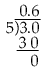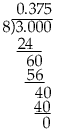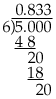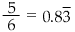Quandaries and Queries I'm trying to help my son work with turning fractions into decimals and this seems foreigh to me after 20 years of not being in school. Can you give me a few illustrations and/or examples of how to do that? Hi, A common fraction can be transformed into its decimal form by long division. For example to express 3/5 in decimal form divide 5 into 3.an hence 3/5 = 0.6 Another example is 3/8and hence 3/8 = 0.375 Often you find a decimal form that repeats, for example 5/6In this case we usually writewhere the bar over the 3 indicates that it repeats. I hope this helps, Penny Go to Math Central